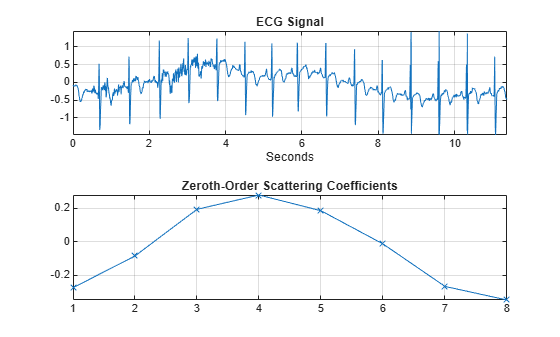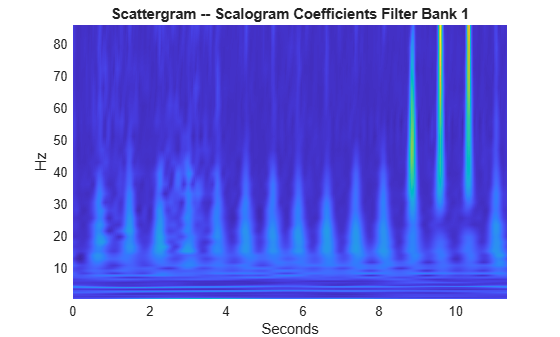Documentation

# scatteringTransform

Wavelet 1-D scattering transform

## Syntax

``s = scatteringTransform(sf,x)``
``[s,u] = scatteringTransform(sf,x)``

## Description

````s = scatteringTransform(sf,x)` returns the wavelet 1-D scattering transform of `x` for the scattering decomposition framework, `sf`. `x` is a real-valued vector or matrix. If `x` is a matrix, `scatteringTransform` applies the scattering transform to each column of `x` separately. `s` is a cell array with Nfb + 1 elements, where Nfb is the number of filter banks in `sf`. The number of filter banks is also equal to the number of elements in the `QualityFactors` value of `sf`. Equivalently, the number of elements in `s` is equal to the number of orders in the scattering decomposition. Each element of `s` is a MATLAB® table.The precision of the scattering coefficients depends on the precision specified in the framework `sf`.```

example

````[s,u] = scatteringTransform(sf,x)` returns `s`, the wavelet 1-D scattering transform, and `u`, the scalogram coefficients for each of the scattering orders. `u` is a cell array with Nfb + 1 elements, where Nfb is the number of filter banks in the scattering framework. The number of filter banks is also equal to the number of elements in the `QualityFactors` value of `sf`. Equivalently, the number of elements in `u` is equal to the number of orders in the scattering decomposition. Each element of `u` is a MATLAB table.The precision of the scalogram coefficients depends on the precision specified in the framework `sf`.```

## Examples

collapse all

This example shows how to return the wavelet 1-D scattering transform of a real-valued signal.

Load an ECG signal sampled at 180 Hz.

```load wecg Fs = 180;```

Create a scattering decomposition framework to apply to the signal. Compute the scattering transform of the signal.

```sf = waveletScattering('SignalLength',numel(wecg),... 'SamplingFrequency',Fs)```
```sf = waveletScattering with properties: SignalLength: 2048 InvarianceScale: 5.6889 QualityFactors: [8 1] Boundary: "periodic" SamplingFrequency: 180 Precision: "double" OversamplingFactor: 0 ```
`[S,U] = scatteringTransform(sf,wecg);`

Plot the signal and the zeroth-order scattering coefficients. Note that the invariance scale is one half the duration of the signal.

```t = [0:length(wecg)-1]/Fs; subplot(2,1,1) plot(t,wecg) grid on axis tight xlabel('Seconds') title('ECG Signal') subplot(2,1,2) plot(S{1}.signals{1},'x-') grid on axis tight title('Zeroth-Order Scattering Coefficients')```Visualize the scattergram for the first-order scalogram coefficients.

```figure scattergram(sf,U,'FilterBank',1)```## Input Arguments

collapse all

Scattering decomposition framework, specified as a `waveletScattering` object.

Input data, specified as a real-valued vector or matrix. If `x` is a vector, the number of samples in `x` must equal the `SignalLength` value of `sf`. If `x` is a matrix, the number of rows in `x` must equal the `SignalLength` value of `sf`.

Data Types: `double` | `single`

## Output Arguments

collapse all

Scattering coefficients, returned as a cell array. `s` is a cell array with `Nfb`+1 elements where Nfb is the number of filter banks in the scattering decomposition framework. Nfb is equal to the number of elements in the `QualityFactors` value of `sf`. Equivalently, the number of elements of `s` is equal to the number of orders in `sf`.

The precision of the scattering coefficients depends on the precision specified in the framework `sf`.

Each element of `s` is a MATLAB table with the following variables:

Scattering coefficients, returned as a cell array. If `x` is a vector, each element of `signals` is a column vector. If `x` has N columns, each element of `signals` is a M-by-N matrix, where M is the number of scattering coefficients.

Data Types: `double` | `single`

Scattering path used to obtain the scattering coefficients, returned as a row vector. Each column of `path` corresponds to one element of the path. The scalar 0 denotes the original signal. Positive integers in the Lth column denote the corresponding wavelet filter in the (L-1)th filter bank. Wavelet bandpass filters are ordered by decreasing center frequency.

Data Types: `double`

Bandwidth of the scattering coefficients, returned as a scalar. If you specify a sampling frequency in the scattering framework, the bandwidth is in hertz. Otherwise, the bandwidth is in cycles/sample.

Data Types: `double`

Base-2 log resolution of the scattering coefficients, returned as a scalar.

Data Types: `double`

Scalogram coefficients, returned as a cell array. `u` is a cell array with Nfb+1 elements, where Nfb is the number of filter banks in the scattering decomposition framework. Nfb is equal to the number of elements in the `QualityFactors` value of `sf`. Equivalently, the number of elements of `u` is equal to the number of orders in `sf`.

The precision of the scalogram coefficients depends on the precision specified in the framework `sf`.

Each element of `u` is a MATLAB table with the following variables:

Scalogram coefficients, returned as a cell array. If `x` is a vector, each element of `coefficients` is a column vector. If `x` has N columns, each element of `coefficients` is a M-by-N matrix, where M is the number of scalogram coefficients.

Data Types: `double` | `single`

Scattering path used to obtain the scalogram coefficients, returned as a row vector. Each column of `path` corresponds to one element of the path. The scalar 0 denotes the original signal. Positive integers in the Lth column denote the corresponding wavelet filter in the (L-1)th filter bank. Wavelet bandpass filters are ordered by decreasing center frequency.

Data Types: `double`

Bandwidth of the scalogram coefficients, returned as a scalar. If you specify a sampling frequency in the scattering decomposition framework, the bandwidth is in hertz. Otherwise, the bandwidth is in cycles/sample.

Data Types: `double`

Base-2 log resolution of the scalogram coefficients, returned as a scalar.

Data Types: `double`

## See Also

#### Introduced in R2018b

##### Support